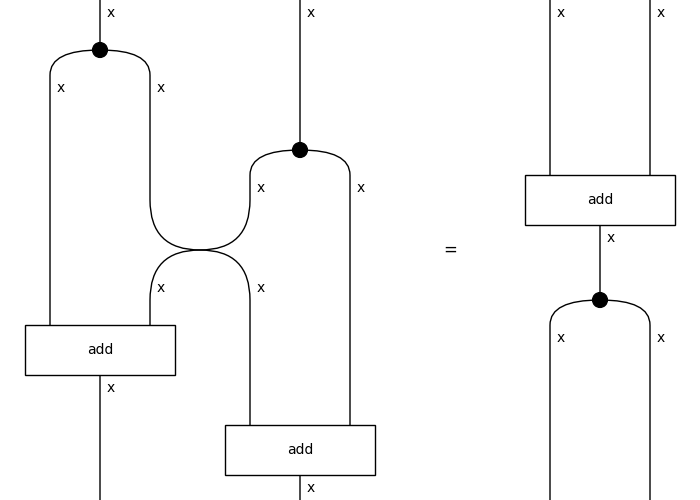# Functor#

class discopy.cartesian.Functor(ob=None, ar=None, cod=None)[source]#

A cartesian functor is a symmetric functor that preserves copies.

Parameters

Example

We build a functor into python functions.

```>>> x = Ty('x')
```>>> from discopy.drawing import Equation